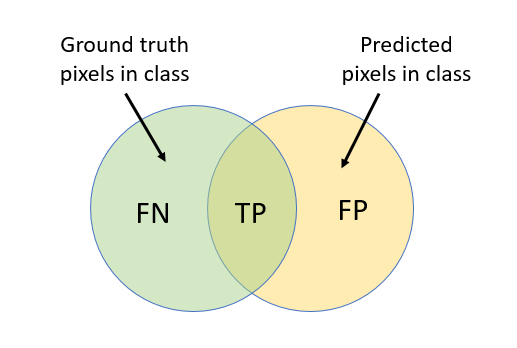Documentation

### This is machine translation

Mouseover text to see original. Click the button below to return to the English version of the page.

# semanticSegmentationMetrics

Semantic segmentation quality metrics

## Description

A `semanticSegmentationMetrics` object encapsulates semantic segmentation quality metrics for a set of images.

## Creation

Create a `semanticSegmentationMetrics` object using the `evaluateSemanticSegmentation` function.

## Properties

expand all

Confusion matrix, specified as a square table. Each table element (i,j) is the count of pixels known to belong to class i but predicted to belong to class j.

Normalized confusion matrix, specified as a square table. Each table element (i,j) is the count of pixels known to belong to class i but predicted to belong to class j, divided by the total number of pixels predicted in class j. Elements are in the range [0, 1].

Semantic segmentation metrics aggregated over the data set, specified as a table. `DataSetMetrics` contains up to five metrics, depending on the value of the `'Metrics'` name-value pair used with `evaluateSemanticSegmentation`:

• `GlobalAccuracy` — Ratio of correctly classified pixels to total pixels, regardless of class.

• `MeanAccuracy` — Ratio of correctly classified pixels in each class to total pixels, averaged over all classes. The value is equal to the mean of `ClassMetrics.Accuracy`.

• `MeanIoU` — Average intersection over union (IoU) of all classes. The value is equal to the mean of `ClassMetrics.IoU`.

• `WeightedIoU` — Average IoU of all classes, weighted by the number of pixels in the class.

• `MeanBFScore` — Average boundary F1 (BF) score of all images. The value is equal to the mean of `ImageMetrics.BFScore`.

Semantic segmentation metrics for each class, specified as a table. `ClassMetrics` contains up to three metrics for each class, depending on the value of the `'Metrics'` name-value pair used with `evaluateSemanticSegmentation`:

• `Accuracy` — Ratio of correctly classified pixels in each class to the total number of pixels belonging to that class according to the ground truth. Accuracy can be expressed as:

`Accuracy` = (TP + TN ) / (TP + TN + FP + FN)

PositiveNegative
PositiveTP: True PositiveFN: False Negative
NegativeFP: False PositiveTN: True Negative

TP: True positives and FN is the number of false negatives.

• `IoU` — Ratio of correctly classified pixels to the total number of pixels that are assigned that class by the ground truth and the predictor. IoU can be expressed as:

`IoU` = TP / (TP + FP + FN)

The image describes the true positives (TP), false positives (FP), and false negatives (FN).• `MeanBFScore` — Boundary F1 score for each class, averaged over all images.

Semantic segmentation metrics for each image in the data set, specified as a table. `ImageMetrics` contains up to five metrics, depending on the value of the `'Metrics'` name-value pair used with `evaluateSemanticSegmentation`:

• `GlobalAccuracy` — Ratio of correctly classified pixels to total pixels, regardless of class

• `MeanAccuracy` — Ratio of correctly classified pixels to total pixels, averaged over all classes in the image

• `MeanIoU` — Average IoU of all classes in the image

• `WeightedIoU` — Average IoU of all classes in the image, weighted by the number of pixels in each class

• `MeanBFScore` — Average BF score of each class in the image

Each image metric returns a vector, with one element for each image in the data set. The order of the rows matches the order of the images defined by the input `PixelLabelDatastore` objects representing the data set.

## Examples

collapse all

The `triangleImages` data set has 100 test images with ground truth labels. Define the location of the data set.

`dataSetDir = fullfile(toolboxdir('vision'),'visiondata','triangleImages');`

Define the location of the test images.

`testImagesDir = fullfile(dataSetDir,'testImages');`

Define the location of the ground truth labels.

`testLabelsDir = fullfile(dataSetDir,'testLabels');`

Create an imageDatastore holding the test images.

`imds = imageDatastore(testImagesDir);`

Define the class names and their associated label IDs.

```classNames = ["triangle","background"]; labelIDs = [255 0];```

Create a pixelLabelDatastore holding the ground truth pixel labels for the test images.

`pxdsTruth = pixelLabelDatastore(testLabelsDir,classNames,labelIDs);`

Load a semantic segmentation network that has been trained on the training images of `noisyShapes`.

```net = load('triangleSegmentationNetwork'); net = net.net;```

Run the network on the test images. Predicted labels are written to disk in a temporary directory and returned as a pixelLabelDatastore.

`pxdsResults = semanticseg(imds,net,"WriteLocation",tempdir);`
```Running semantic segmentation network ------------------------------------- * Processing 100 images. * Progress: 100.00% ```

Evaluate the prediction results against the ground truth.

`metrics = evaluateSemanticSegmentation(pxdsResults,pxdsTruth);`
```Evaluating semantic segmentation results ----------------------------------[==================================================] 100% Elapsed time: 00:00:02 Estimated time remaining: 00:00:00 * Finalizing... Done. * Data set metrics: GlobalAccuracy MeanAccuracy MeanIoU WeightedIoU MeanBFScore ______________ ____________ _______ ___________ ___________ 0.90624 0.95085 0.61588 0.87529 0.40652 ```

Display the classification accuracy, the intersection over union, and the boundary F-1 score for each class.

`metrics.ClassMetrics`
```ans=2×3 table Accuracy IoU MeanBFScore ________ _______ ___________ triangle 1 0.33005 0.028664 background 0.9017 0.9017 0.78438 ```